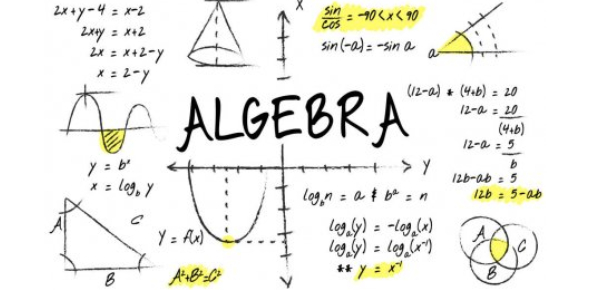# Math Algebra Quiz For 13-14 Years Student

10 Questions | Attempts: 12494SettingsGet ready to take our Math algebra quiz for 13-14 years students. This is to test 13 - 14 years old on their algebra knowledge. If you are at school or even if you have passed it, algebra is something you must have studied. Here, you need to show how well you understand or know algebra. Score 80 or above on this quiz, and you are an expert in it.

• 1.
Expand : 5(12c + 7) - (1 - 55c)
• A.

60c + 36 -1 + 55c = 115c + 135

• B.

60c + 36 -1 + 55c

• C.

55c = 115c + 34

• D.

60c + 35 - 1 + 55c = 115c + 34

• 2.
Algebra is the study of triangles.
• A.

True

• B.

False

• 3.
Find x 2x + 3 = 7x - 3
• A.

5x = 3

• B.

1.6 = x

• C.

3c + 3c = 7x - 2x 6 = 5x 6/5 = x 1.2 = x

• D.

4x = 3

• 4.
This sum is incorrect : 3-3+8-5x = 13x
• A.

True

• B.

False

• 5.
Trigonometry is also called algebra.
• A.

True

• B.

False

• 6.
000.33333333330000 *109
• A.

3.3

• B.

333333333.3

• C.

333333330000

• D.

None of these

• 7.
An ice cream costs c pence and a pizza costs d pence. Write down an expression for the cost of 2 ice creams and 2 pizzas.
• A.

1d + 1c

• B.

2d

• C.

2c + 2d

• D.

2c

• 8.
Algebra is only usable in geometry.
• A.

True

• B.

False

• 9.
A car costs a pence and a bike costs b pence. Write down an expression that defines the cost of 2 cars and 2 bikes.
• A.

2a + 2b

• B.

4a + 2b

• C.

2a + 2a

• D.

None of these

• 10.
4m+5+2m-1 This expression can be simplified as 6m + 6.
• A.

True

• B.

False

## Related TopicsBack to top
×

Wait!
Here's an interesting quiz for you.